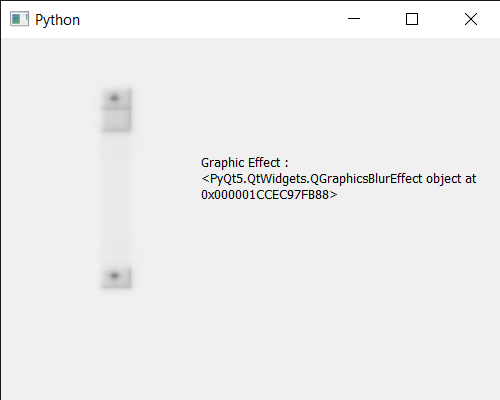Open in App
Not now

# PyQt5 QScrollBar – Getting Graphic Effect of it

• Last Updated : 05 Jan, 2023

In this article, we will see how we can get graphic effect of the QScrollBar. QScrollBar is a control that enables the user to access parts of a document that is larger than the widget used to display it. Slider is the scroll-able object inside the bar. The setGraphicsEffect function is for setting the scroll bar’s graphics effect. Sets effect as the widget’s effect. If there already is an effect installed on the scroll bar, QWidget will delete the existing effect before installing the new effect. It can be set with the help of setGraphicsEffect method.

In order to do this we will use graphicsEffect method with the scroll bar object. Syntax : scroll.graphicsEffect() Argument : It takes no argument Return : It returns QGraphicsEffect object

Below is the implementation

## Python3

 `# importing libraries` `from` `PyQt5.QtWidgets ``import` `*` `from` `PyQt5 ``import` `QtCore, QtGui` `from` `PyQt5.QtGui ``import` `*` `from` `PyQt5.QtCore ``import` `*` `import` `sys`     `class` `Window(QMainWindow):`   `    ``def` `__init__(``self``):` `        ``super``().__init__()`   `        ``# setting title` `        ``self``.setWindowTitle("Python ")`   `        ``# setting geometry` `        ``self``.setGeometry(``100``, ``100``, ``500``, ``400``)`   `        ``# calling method` `        ``self``.UiComponents()`   `        ``# showing all the widgets` `        ``self``.show()`       `    ``# method for components` `    ``def` `UiComponents(``self``):`   `        ``scroll ``=` `QScrollBar(``self``)`   `        ``# setting geometry of the scroll bar` `        ``scroll.setGeometry(``100``, ``50``, ``30``, ``200``)`   `        ``# making its background color to green` `        ``scroll.setStyleSheet("background : lightgrey;")`   `        ``# QGraphicsEffect` `        ``graphic ``=` `QGraphicsBlurEffect(``self``)` `        `  `        ``# setting graphic effect to the scroll bar` `        ``scroll.setGraphicsEffect(graphic)`   `        ``# creating a label` `        ``label ``=` `QLabel("GeeksforGeeks", ``self``)`   `        ``# setting geometry to the label` `        ``label.setGeometry(``200``, ``100``, ``300``, ``80``)`   `        ``# making label multi line` `        ``label.setWordWrap(``True``)`   `        ``# getting value changed signal` `        ``scroll.valueChanged.connect(``lambda``: do_action())`   `        ``# method called when signal is emitted` `        ``def` `do_action():` `            `  `            ``# setting text to the label` `            ``label.setText("Current Value : " ``+` `str``(scroll.value()))`   `        ``# getting graphic effect of scroll bar` `        ``value ``=` `scroll.graphicsEffect()`   `        ``# setting text to the label` `        ``label.setText("Graphic Effect : " ``+` `str``(value))`     `# create pyqt5 app` `App ``=` `QApplication(sys.argv)`   `# create the instance of our Window` `window ``=` `Window()`   `# start the app` `sys.exit(App.``exec``())`

Output :My Personal Notes arrow_drop_up
Related Articles